# How to Teach Multiplication — 7 Simple Strategies for Success

Wondering how to teach multiplication? Tired of drills? We've put together seven awesome strategies that get results every time. You can thank us later.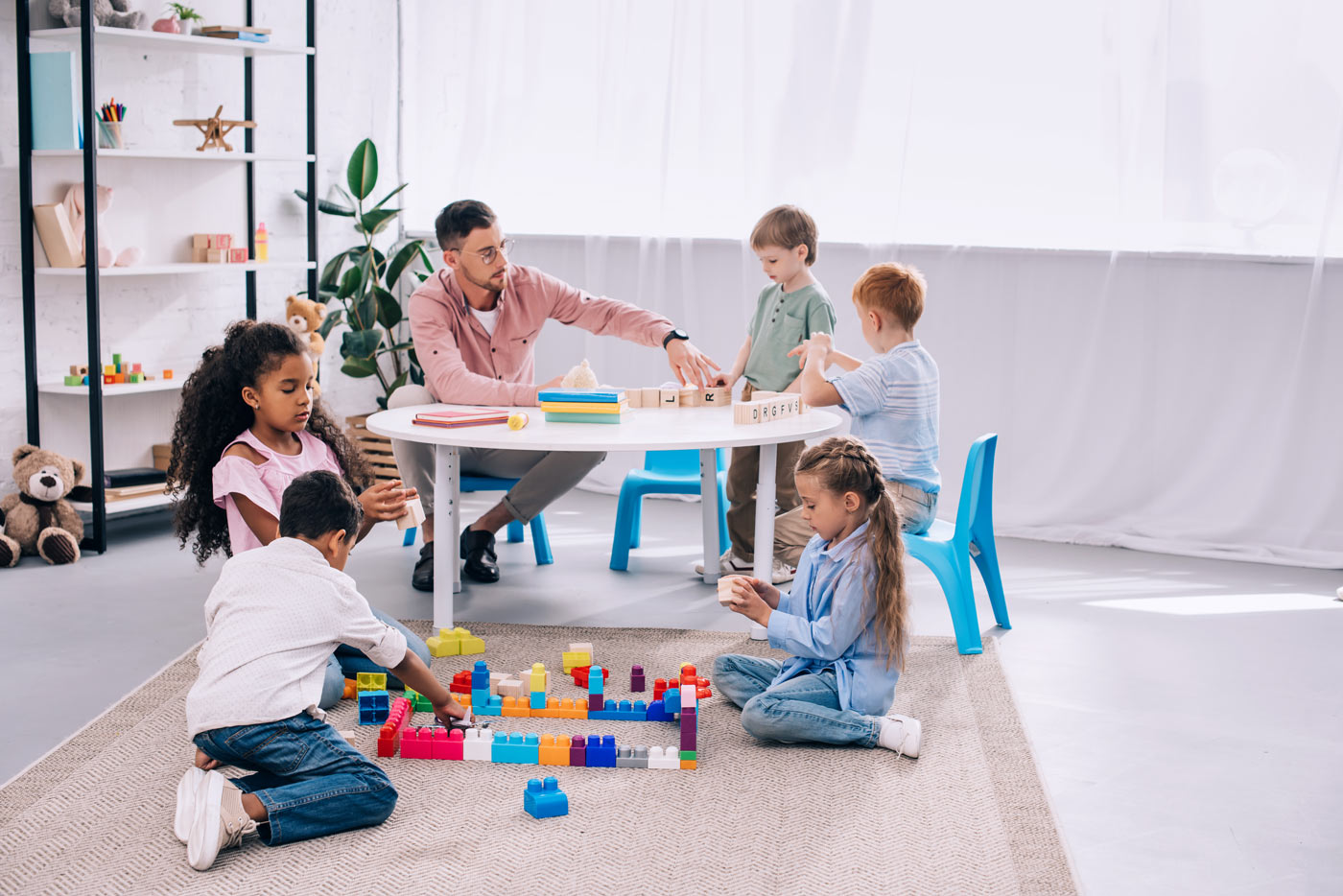Math Minds

Thursday, 28th July 2022

Learning multiplication can be one of the most challenging math concepts for students to grasp. It is also one of the most fundamental skills, as it lays the foundation for so many more advanced ideas.

Fortunately, there are a number of strategies that teachers (and parents) can use to help their kids succeed. In this article, we share seven secrets—read on to discover the best ways to teach multiplication.

Before you can even begin to teach multiplication, your students need to have a strong understanding of the basic concepts of addition and subtraction. Below are five vital maths strategies that kids should first master before they can even think about attempting multiplication or repeated addition for that matter.

### Number Bonds

The number bond for 7 is: 0 + 7, 1 + 6, 2 + 5, 3 + 4. Every number from 1 to 10 has a number bond. Teaching multiplication strategies makes little sense until these have been mastered.

### Doubling

Students must first learn what it means to double a number before they can move on to grouping. Make sure they can calculate 1+1 all the way up to 24+24. Herein also lies an important skill—partitioning numbers. To teach 18+18 for example, we can think of 10+10+8+8 = 20 + 16 = 36.

### Halving

Like doubling, halving is a fundamental concept that we think students must understand before they can move on to multiplication.

### Friends of ten

Let's imagine we're adding 7 to 14 mentally (without using our fingers or counting up by ones). Students should be familiar with the idea that 14+6=20, and since we are adding 7, the answer therefore must be 20+1=21

### Compensation

Another approach to our 7+14 example involves rounding an addend and then adjusting (compensating) the answer accordingly. Given that 7 is only 3 less than 10, we could have added 10+14=24 and then subtracted 3 to give us 21.

## 2. Use a variety of models and manipulatives

There are a number of different ways to model multiplication, and using a variety of physical manipulatives is a great way to help your students understand the concept. Some popular models include: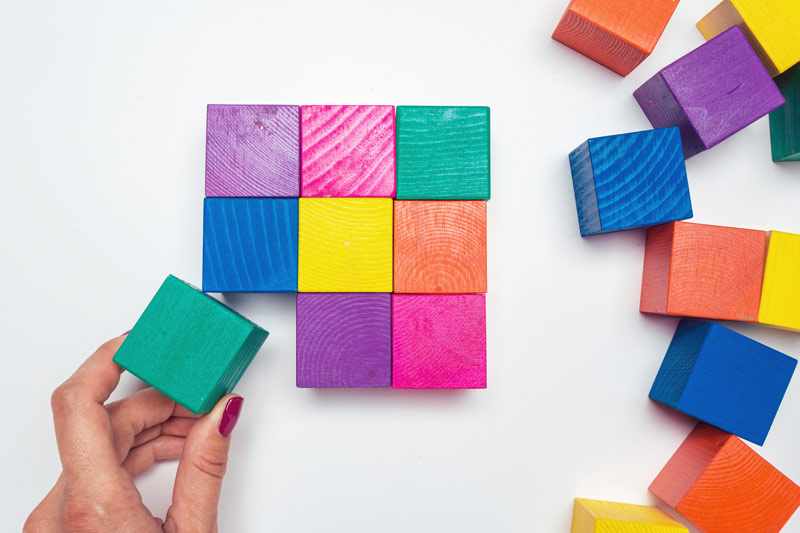### Grouping items

Using blocks or even just a simple pile of objects, have students group the items into equal piles. This will help them visualize the concept of equal groups and the underlying notion that "x" groups of "y" gives you a number, "z".

### Array and Area Models

An array is simply a rectangular arrangement of objects in rows and columns and is a great way to teach multiplication visually. Area models are a variation of this where the number of rows and columns are represented by measurements, rather than just groups of objects.

### Number Lines

A number line is a visual way to show addition and subtraction, but it can also be used to introduce skip counting. Students would start at 0 and count up by the number they are multiplying by. So, if we wanted to model 3 x 4 using a number line, we would start at 0 and count up by 3 four times (0, 3, 6, 9, 12).

### Drawing pictures to represent multiplication problems

This is a great strategy for young struggling students who are just learning the basics of multiplication. Using drawings to represent a multiplication sentence can help students visualize the concept and understand what they are doing.

## 3. Highlight the core properties

To help students learn their tables, it is important that they understand certain math facts and are able to use the various properties of multiplication.

### Commutative Property

The commutative property states that the order of the numbers being multiplied does not change the product. So, 3 x 5 will always equal 5 x 3. To bring home this point, you could have students draw out an array for each and then compare the answers. 3 x 5 = 15 and 5 x 3 = 15.

This realisation is epic for many students, as they discover that every multiplication problem is actually two problems in disguise!

### Associative Property

The associative property states that we can regroup numbers when multiplying and the product will stay the same. 2 x 4 x 3 could be thought of as (2 x 4) x 3 or 2 x (4 x 3).

### Multiplication and division are opposites

Many educators teach multiplication facts in isolation from division problems. They see it as a distinct concept. However, we believe that multiplication should be taught alongside division (and vice versa) as they are intimately related.

Help your students to make connections by thinking of them in 'families'. 3, 4 and 12 are a family combo. If students are learning 3 x 4 = 12. They should be writing down 12 ÷ 3 = 4 and 12 ÷ 4 = 3 at the same time.

### Distributive Property

The distributive property is a little more complicated, but it's a key concept for students to understand, especially for those in third grade and above. It states that when we multiply a number by a group of numbers, we can multiply that number by each number in the group and then add the products together.

If we wanted to find 6 x 14, we could break it down into 6 x (10 + 4). This would give us 60 + 24, which is equal to 84.

## 4. Teach multiplication facts in a strategic order

• Any number multiplied by one remains the same.

• Any number multiplied by two is the same as doubling that number, or in other words, that number plus itself. 7 x 2 = 7 + 7.

• Any number multiplied by 10 — put a zero on the end.

• Any number multiplied by 11 is the same digit repeated twice (works only up till 9).

That's already a large chunk of the multiplication table taken care of. From here we move to mastery of the 3, 4 and 5 times tables.

### How to teach the 3, 4s and 5s

• The threes: Double a number and then add one more group. For instance to calculate 3 x 6. We know 2 x 6 = 12, so we add one more group of 6 to 12 to arrive at 18.

• The fours: Since students already understand the 2 x tables and are fluent in doubling numbers, we can take a number sense approach—double the product of your 2 times table to understand the 4s. For example, in order to calculate 4 x 7, we can think of 2 x 7 = 14, and then double that. So 14 x 2 = 28.

• The fives: All products in the 5 times table end in either 0 or 5. One simple tip is to first ensure students can halve all the numbers in the 10 x tables. This is also an effective way to introduce them to the concept of halving larger numbers. To calculate 5 x 7, we first think of 10 groups of 7 (which is 70) and then half that as we only need 5 groups of 7, leaving us with 35.

### The trick for the 9s

At Math Minds our students master the nines in under 5 minutes. Once kids are fluent in the number bonds for 9, teaching them the 9 x tables is a cakewalk. Of course, you could use the 'finger trick' but we've found greater success with the following multiplication strategy.

First get students to write all number bonds out for 9 vertically. 1+8, 2+7, 3+6 ... etc. Ask them if they notice any patterns. Establish that they unknowingly wrote out the entire 9 times tables!

So, to calculate 9 x 7 the first digit of their answer will always be one less than the number they are multiplying with 9. In this case, one less than 7 is 6. The second digit will be 6's number bond (partner) which is 3. So the answer is 63. Let's go!

### The 'milestone' strategy to master everything else

Once students are ready to learn their times tables for 6, 7, 8 and 12 we get them to think about using the multiples of 5 and 10 as stepping stones to fill out the rest. This approach centres around understanding rather than rote practice. It's also much more efficient than skip counting by starting from 0.

Let's take a common multiplication fact that a lot of kids struggle with, 6 x 8. Check out this approach below using our 5 and 10 multiplication tables as the jump-off:

• In order to calculate 6 groups of 8, we first think about 5 groups of 8, which is 40. We then only need to add one more group of 8. So 40 + 8 = 48.

• Alternatively, we could start with 10 groups of 6, which is 60, as our starting point. Because we're calculating 8 groups of 6, we must subtract 2 groups of 6 from 60. So we do 60 - (2 x 6) = 60 - 12 = 48.

## 5. Bring the concepts into daily life

When you're teaching multiplication equations, look for opportunities to incorporate the concepts into everyday life experiences. This will depend on the age of your students and their development along the Concrete-Pictorial-Abstract continuum.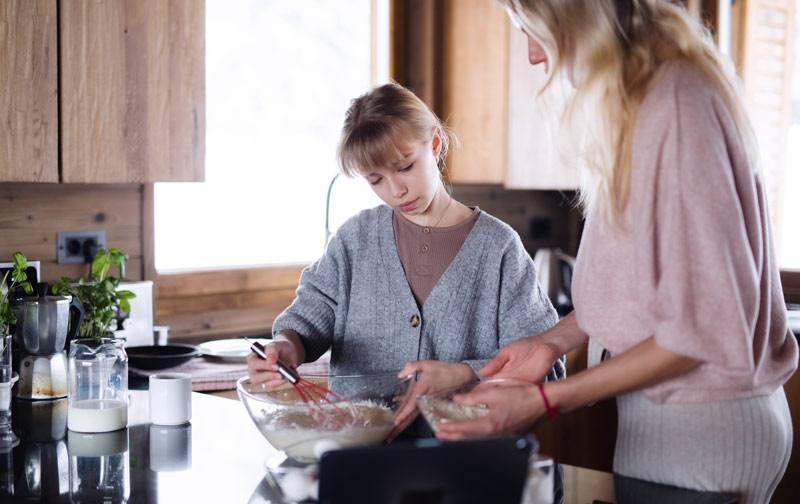### Concrete strategies

At this initial level, students need lots of hands-on experiences to help them make sense of the concept. One way to provide this is with concrete manipulatives such as counters, beads, or blocks.

### Pictorial Phase

Here students begin to understand that multiplication is a way of representing repeated addition. They can use pictures and drawings to represent multiplication problems. For example, they might draw 5 rows of 4 dots to represent 5 x 4.

### Abstract Phase

By this stage, students are able to use symbols to represent multiplication. They understand that the product of two numbers is the answer to a multiplication problem, regardless of how the problem is represented. For example, they know that 4 x 5 can be represented as 4 + 4 + 4 + 4 + 4, as 5 + 5 + 5 + 5, or simply as 20.

### Real-world examples

No matter which stage they're at, students should see the connection between multiplication and life around them with real-world scenarios. This makes the learning meaningful. It helps them experience their surroundings through the lens of mathematics. Here are some great ideas for bringing multiplication to life:

• Have students double or triple a cooking recipe.

• Measure ingredients using different units to see how they convert.

• Plant a garden and have students calculate how many seeds to plant, based on the amount of space they have.

• Tally how many of each type of animal or object they see (e.g. the number of dogs, trees, stop signs).

• When shopping, have students compare different brands of the same product to see which is the better deal.

• Count the number of items needed for a party.

• Calculate the cost of painting a house or ordering food from a menu.

Each of these activities provides opportunities for students to practice their multiplication skills in a way that is relevant and relatable to their lives. If you want to help them get better at maths, they should see that multiplication is more than just a bunch of abstract numbers that exist during class—It's a tool they can use every day!

## 6. Make learning multiplication fun

Whenever possible, stay away from 'drills' and repetition. While it may have its place in some circumstances, this type of practice is often boring and ineffective in the early stages.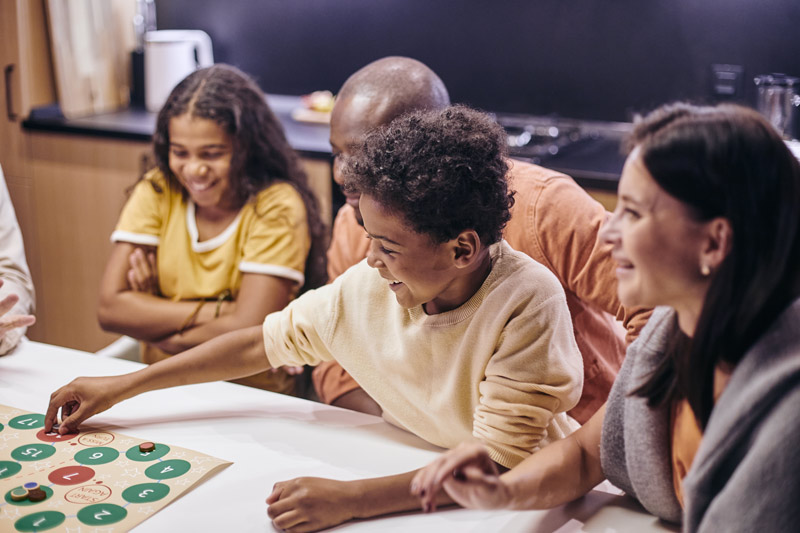Furthermore, teaching children to recite or practice skip counting might appear as a good way to help them learn their tables—but this isn't always the case. In fact, it can often do more harm than good.

This is because when children focus on memorizing a sequence of numbers, like 3, 6, 9, 12 etc. they're usually not understanding what they're doing. So when they encounter a problem that doesn't fit the pattern they're used to, they can get easily confused and frustrated.

A much better way to help children learn multiplication is to let them explore the concepts through a variety of activities that build a number sense.

Use estimation. Play with patterns. Group and regroup objects. Get visual in representing multiplication problems. Allow them to explore and develop connections. As they do this, they'll gradually start to see relationships between numbers—Which is exactly what multiplication is all about!

### Learn through play

Here are some effective and fun ways for teaching multiplication:

• Play 'guess my number', using multiplication combos.

• Create a multiplication chart and look for patterns.

• Write a story problem.

• Make multiplication strategies posters.

• Draw pictures to represent multiplication word problems.

• Play multiplication bingo or guess who.

• Design a multiplication board game.

Remember, the goal is to help your students develop a strong understanding of what multiplication is, why it's important and how it works. So, don't be afraid to get creative! Try to come up with as many different strategies and ways to explain the concepts as possible. The more variety, the better!

### Harness technology

In today's world, there's no excuse for lessons to be boring and outdated. With all of the amazing resources out there, it's easy to make learning multiplication interactive, engaging and fun! Here are some great ideas:

• Watch a multiplication rap video on YouTube.

• Complete an online quiz.

• Play multiplication games on a website.

• Make a multiplication game on Scratch.

• Try out a multiplication app on a tablet or smartphone.

Some of the best maths apps for kids can be used to support the teaching of multiplication facts. They often include games, activities and challenges that can be adapted to different levels, which makes them perfect tools for differentiated learning!

## 7. Focus on understanding before memorization

It's easy to get caught up in the idea that children need to memorize their multiplication facts as quickly as possible. But it's important to remember that memorization without understanding is futile. In fact, it's often one of the main reasons why children struggle with mathematics later on in life.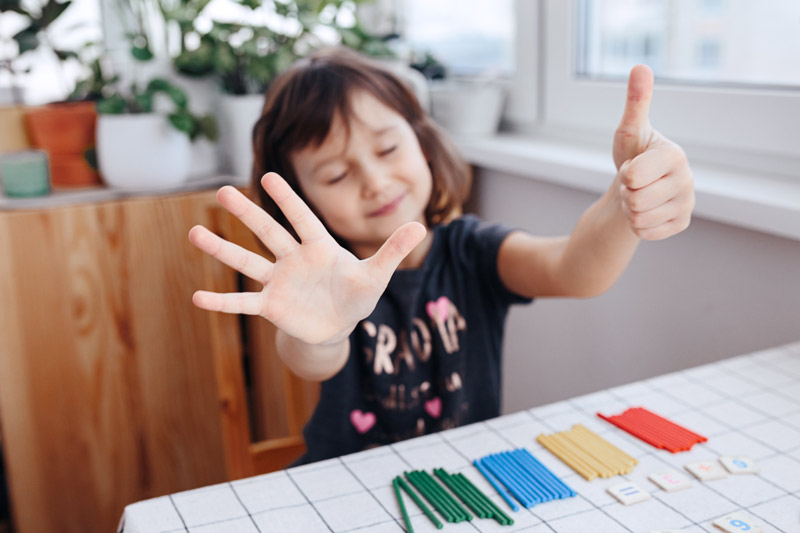The aim is to focus on understanding, helping your students develop a deep conceptual fluency. As a parent or educator make sure you follow these 3 guiding principles:

### 1. Use the correct language of multiplication

Equip students with the right vocabulary associated with multiplication: lots of, groups of, times, multiply, multiplied by, multiple of, factor, product, once, twice, three times etc. Vary your questioning technique:

• What is 3 multiplied by 9?

• Give me six multiples of 8.

• What is the product of 7 and 5?

• Calculate 6 times 12.

• Is 7 a factor of 14?

• What is 35 divided by 5?

• Three less than double of 17.

This is where your teaching methods need to adapt according to the child you're working with. Some children will understand multiplication much quicker than others and be prepared that different mental strategies will work with different children. The key is to be flexible with your lesson.

In our maths tutoring sessions, we personalise our approach according to each individual student's learning style and needs—we teach strategies that fit with how you think and learn best.

### Encourage mastery

Encourage students to keep practising their multiplication facts until they have mastered them. This doesn't mean drilling them over and over again until they're sick of the sight of numbers! It simply means providing opportunities for them to revisit, revise and practise in numerous ways.

## Final Thoughts

When it comes to learning how to teach multiplication, there is no one-size-fits-all solution. There are so many teaching resources out there and you might even find other teachers who are proponents of rote repetition.

Experiment to find an approach that works for you and your students. We can guarantee one thing though. By focusing on even just a few of these 7 strategies, you can be confident that your students will not only develop a strong conceptual understanding of multiplication, but they'll also be able to apply it successfully in their everyday lives.

When you teach students make sure they are engaged and enjoying the discovery! Multiplication shouldn't be a chore. By using the right combination of techniques, you can turn it into an exciting learning experience that your students will never forget!

## Catch up, keep up and get ahead

### In-Center or Online

In less than one hour we'll identify your strengths, knowledge gaps and tailor a customised learning plan. Ready to go?

Free Assessment